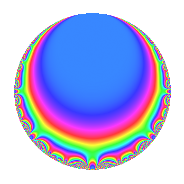# Properties

 Label 28.2.eLevel 28 Weight 2 Character orbit e Rep. character $$\chi_{28}(9,\cdot)$$ Character field $$\Q(\zeta_{3})$$ Dimension 2 Newforms 1 Sturm bound 8 Trace bound 0

# Related objects

## Defining parameters

 Level: $$N$$ = $$28 = 2^{2} \cdot 7$$ Weight: $$k$$ = $$2$$ Character orbit: $$[\chi]$$ = 28.e (of order $$3$$ and degree $$2$$) Character conductor: $$\operatorname{cond}(\chi)$$ = $$7$$ Character field: $$\Q(\zeta_{3})$$ Newforms: $$1$$ Sturm bound: $$8$$ Trace bound: $$0$$

## Dimensions

The following table gives the dimensions of various subspaces of $$M_{2}(28, [\chi])$$.

Total New Old
Modular forms 14 2 12
Cusp forms 2 2 0
Eisenstein series 12 0 12

## Trace form

 $$2q - q^{3} - 3q^{5} - 4q^{7} + 2q^{9} + O(q^{10})$$ $$2q - q^{3} - 3q^{5} - 4q^{7} + 2q^{9} + 3q^{11} + 4q^{13} + 6q^{15} - 3q^{17} + q^{19} - q^{21} - 3q^{23} - 4q^{25} - 10q^{27} - 12q^{29} + 7q^{31} + 3q^{33} + 15q^{35} + q^{37} - 2q^{39} + 12q^{41} - 8q^{43} + 6q^{45} + 9q^{47} + 2q^{49} - 3q^{51} - 3q^{53} - 18q^{55} - 2q^{57} - 9q^{59} + q^{61} - 10q^{63} - 6q^{65} + 7q^{67} + 6q^{69} + q^{73} - 4q^{75} + 3q^{77} + 13q^{79} - q^{81} + 24q^{83} + 18q^{85} + 6q^{87} - 15q^{89} - 8q^{91} + 7q^{93} + 3q^{95} - 20q^{97} + 12q^{99} + O(q^{100})$$

## Decomposition of $$S_{2}^{\mathrm{new}}(28, [\chi])$$ into irreducible Hecke orbits

Label Dim. $$A$$ Field CM Traces $q$-expansion
$$a_2$$ $$a_3$$ $$a_5$$ $$a_7$$
28.2.e.a $$2$$ $$0.224$$ $$\Q(\sqrt{-3})$$ None $$0$$ $$-1$$ $$-3$$ $$-4$$ $$q-\zeta_{6}q^{3}+(-3+3\zeta_{6})q^{5}+(-1-2\zeta_{6})q^{7}+\cdots$$